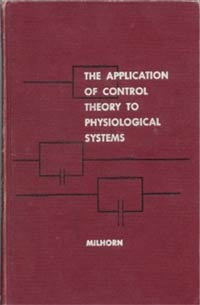#####CLICK ON A STORE TO ORDER YOUR COPY: Alibris Biblio.com

Books by H. Thomas Milhorn

The Application of Control Theory to Physiological Systems
ISBN #66-156231966
x

It has been known for many years that homeostatic mechanisms maintain a constant internal environment. The constancy of this environment is maintained by various control systems which employ what is known as negative feedback. Control theory, for instance, helps to explain how a system such as body temperature regulation takes place -- how the body can always return its temperature to the same value regardless of the direction of the initial change.

One danger which must be recognized in applying control theory to biological systems is that the researcher should be certain that he or she is doing just that and not the opposite; that is, attempting to apply physiological systems to control theory.

This book is an outgrowth of a desire that the author had during his tour in graduate school--that of a text which combined engineering principles with physiological applications. It assumes that the student has had mathematics through calculus and general physics. The material in the text comprises a one semester course, which has the same name as the title of this book, which was taught by the author in the Department of Physiology and Biophysics at the University of Mississippi Medical Center.

Chapter 1
The solution of ordinary linear differential equations by the classical method

Chapter 2
Partial fraction expansion, the Laplace transformation and the Laplace transform solution of ordinary linear differential equations

Chapter 3
Obtaining the technological system differential equations

Chapter 4
Obtaining the physiological system differential equations

Chapter 5
Analog computer programming

Chapter 6
Transient analysis of physical systems

Chapter 7
An introduction to technological control systems

Chapter 8
An introduction to physiological control systems

Chapter 9
Block diagram manipulation, signal flow graphs and symbol-and-arrow diagrams

Chapter 10
Steady-state errors in feedback control systems

Chapter 11
Static characteristics of Technological and physiological control systems

Chapter 12
The application of frequency analysis methods to technological and physiological systems

Chapter 13
The root-locus plot

Chapter 14
The application of control theory to a disease state (Chapter Cheyne-Stokes breathing)

Chapter 15
The respiratory system

Chapter 16
Human eye tracking of aperiodic functions

Chapter 17
The stretch reflex in human muscle system

Chapter 18
The cardiovascular system

Chapter 19
Further applications of control theory to physiological systems